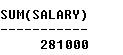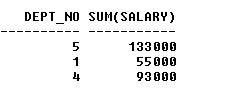## SUM (SQL)

SUM (SQL) function is used to return a total on the values of a column for a group of rows. The SUM (SQL) function adds up all the values for the expression passed to it as an argument, either a column name or the result of a calculation. The value of an argument must be numeric to use the SUM (SQL) function. The SUM (SQL) function cannot be used on columns having a data type other than numeric, such as character or date.

The basic syntax is as follows:

Example 1:

The following SUM SQL will return total amount of salary paid to employees

SELECT sum(salary) FROM employee;

Output:Example 2: